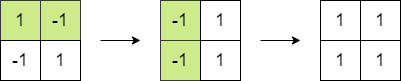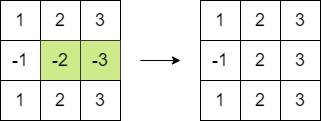Formatted question description: https://leetcode.ca/all/1975.html

# 1975. Maximum Matrix Sum

Medium

## Description

You are given an n x n integer matrix. You can do the following operation any number of times:

• Choose any two adjacent elements of matrix and multiply each of them by -1.

Two elements are considered adjacent if and only if they share a border.

Your goal is to maximize the summation of the matrix’s elements. Return the maximum sum of the matrix’s elements using the operation mentioned above.

Example 1:Input: matrix = [[1,-1],[-1,1]]

Output: 4

Explanation: We can follow the following steps to reach sum equals 4:

• Multiply the 2 elements in the first row by -1.
• Multiply the 2 elements in the first column by -1.

Example 2:Input: matrix = [[1,2,3],[-1,-2,-3],[1,2,3]]

Output: 16

Explanation: We can follow the following step to reach sum equals 16:

• Multiply the 2 last elements in the second row by -1.

Constraints:

• n == matrix.length == matrix[i].length
• 2 <= n <= 250
• -10^5 <= matrix[i][j] <= 10^5

## Solution

To get the maximum matrix sum, at most one element can be negative after performing the operation any number of times. If there is no element 0 and the number of negative elements is odd, then there has to be one negative element. Otherwise, all elements can be non-negative. If there has to be one negative element, it should have the minimum absolute value. Therefore, find the element with the minimum absolute value and make it negative if there has to be one negative element (the element can be positive in the original matrix), and calculate the sum of elements in the matrix.

class Solution {
public long maxMatrixSum(int[][] matrix) {
long sum = 0;
int minPositive = Integer.MAX_VALUE;
int minNegative = Integer.MIN_VALUE;
int positiveCount = 0, negativeCount = 0, zeroCount = 0;
int side = matrix.length;
for (int i = 0; i < side; i++) {
for (int j = 0; j < side; j++) {
int num = matrix[i][j];
sum += Math.abs(num);
if (num > 0) {
minPositive = Math.min(minPositive, num);
positiveCount++;
} else if (num < 0) {
minNegative = Math.max(minNegative, num);
negativeCount++;
} else
zeroCount++;
}
}
if (zeroCount > 0 || negativeCount % 2 == 0)
return sum;
if (positiveCount == 0)
return sum += minNegative * 2;
int minPositiveAbs = minPositive, minNegativeAbs = -minNegative;
sum -= Math.min(minPositiveAbs, minNegativeAbs) * 2;
return sum;
}
}